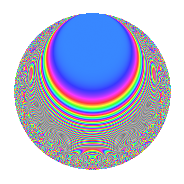# Properties

 Label 1155.2.l.fLevel 1155 Weight 2 Character orbit 1155.l Analytic conductor 9.223 Analytic rank 0 Dimension 40 CM No

# Learn more about

## Newspace parameters

 Level: $$N$$ = $$1155 = 3 \cdot 5 \cdot 7 \cdot 11$$ Weight: $$k$$ = $$2$$ Character orbit: $$[\chi]$$ = 1155.l (of order $$2$$ and degree $$1$$)

## Newform invariants

 Self dual: No Analytic conductor: $$9.22272143346$$ Analytic rank: $$0$$ Dimension: $$40$$ Sato-Tate group: $\mathrm{SU}(2)[C_{2}]$

## $q$-expansion

The dimension is sufficiently large that we do not compute an algebraic $$q$$-expansion, but we have computed the trace expansion.

 $$\operatorname{Tr}(f)(q) =$$ $$40q$$ $$\mathstrut +\mathstrut 4q^{2}$$ $$\mathstrut -\mathstrut 4q^{3}$$ $$\mathstrut +\mathstrut 44q^{4}$$ $$\mathstrut +\mathstrut 4q^{6}$$ $$\mathstrut +\mathstrut 12q^{8}$$ $$\mathstrut -\mathstrut 2q^{9}$$ $$\mathstrut +\mathstrut O(q^{10})$$ $$\operatorname{Tr}(f)(q) =$$ $$40q$$ $$\mathstrut +\mathstrut 4q^{2}$$ $$\mathstrut -\mathstrut 4q^{3}$$ $$\mathstrut +\mathstrut 44q^{4}$$ $$\mathstrut +\mathstrut 4q^{6}$$ $$\mathstrut +\mathstrut 12q^{8}$$ $$\mathstrut -\mathstrut 2q^{9}$$ $$\mathstrut +\mathstrut 6q^{11}$$ $$\mathstrut +\mathstrut 8q^{12}$$ $$\mathstrut +\mathstrut 2q^{15}$$ $$\mathstrut +\mathstrut 68q^{16}$$ $$\mathstrut -\mathstrut 40q^{18}$$ $$\mathstrut +\mathstrut 2q^{21}$$ $$\mathstrut -\mathstrut 4q^{22}$$ $$\mathstrut +\mathstrut 12q^{24}$$ $$\mathstrut -\mathstrut 40q^{25}$$ $$\mathstrut +\mathstrut 32q^{27}$$ $$\mathstrut +\mathstrut 24q^{29}$$ $$\mathstrut -\mathstrut 8q^{31}$$ $$\mathstrut -\mathstrut 52q^{32}$$ $$\mathstrut +\mathstrut 28q^{33}$$ $$\mathstrut +\mathstrut 32q^{34}$$ $$\mathstrut -\mathstrut 40q^{35}$$ $$\mathstrut -\mathstrut 48q^{37}$$ $$\mathstrut -\mathstrut 66q^{39}$$ $$\mathstrut +\mathstrut 16q^{41}$$ $$\mathstrut -\mathstrut 32q^{44}$$ $$\mathstrut +\mathstrut 32q^{48}$$ $$\mathstrut -\mathstrut 40q^{49}$$ $$\mathstrut -\mathstrut 4q^{50}$$ $$\mathstrut +\mathstrut 14q^{51}$$ $$\mathstrut +\mathstrut 72q^{54}$$ $$\mathstrut +\mathstrut 8q^{55}$$ $$\mathstrut +\mathstrut 24q^{57}$$ $$\mathstrut -\mathstrut 80q^{58}$$ $$\mathstrut +\mathstrut 24q^{60}$$ $$\mathstrut -\mathstrut 48q^{62}$$ $$\mathstrut +\mathstrut 76q^{64}$$ $$\mathstrut -\mathstrut 4q^{65}$$ $$\mathstrut -\mathstrut 48q^{67}$$ $$\mathstrut +\mathstrut 32q^{68}$$ $$\mathstrut -\mathstrut 20q^{69}$$ $$\mathstrut -\mathstrut 4q^{70}$$ $$\mathstrut -\mathstrut 128q^{72}$$ $$\mathstrut +\mathstrut 4q^{75}$$ $$\mathstrut +\mathstrut 8q^{77}$$ $$\mathstrut -\mathstrut 28q^{78}$$ $$\mathstrut +\mathstrut 50q^{81}$$ $$\mathstrut -\mathstrut 32q^{82}$$ $$\mathstrut +\mathstrut 24q^{83}$$ $$\mathstrut +\mathstrut 24q^{84}$$ $$\mathstrut +\mathstrut 32q^{87}$$ $$\mathstrut -\mathstrut 16q^{88}$$ $$\mathstrut -\mathstrut 8q^{90}$$ $$\mathstrut -\mathstrut 4q^{91}$$ $$\mathstrut -\mathstrut 20q^{93}$$ $$\mathstrut +\mathstrut 12q^{95}$$ $$\mathstrut -\mathstrut 12q^{96}$$ $$\mathstrut +\mathstrut 100q^{97}$$ $$\mathstrut -\mathstrut 4q^{98}$$ $$\mathstrut +\mathstrut 56q^{99}$$ $$\mathstrut +\mathstrut O(q^{100})$$

## Embeddings

For each embedding $$\iota_m$$ of the coefficient field, the values $$\iota_m(a_n)$$ are shown below.

For more information on an embedded modular form you can click on its label.

Label $$a_{2}$$ $$a_{3}$$ $$a_{4}$$ $$a_{5}$$ $$a_{6}$$ $$a_{7}$$ $$a_{8}$$ $$a_{9}$$ $$a_{10}$$
1121.1 −2.72154 −1.44946 0.948193i 5.40675 1.00000i 3.94475 + 2.58054i 1.00000i −9.27160 1.20186 + 2.74873i 2.72154i
1121.2 −2.72154 −1.44946 + 0.948193i 5.40675 1.00000i 3.94475 2.58054i 1.00000i −9.27160 1.20186 2.74873i 2.72154i
1121.3 −2.50847 1.71957 0.207558i 4.29243 1.00000i −4.31349 + 0.520654i 1.00000i −5.75050 2.91384 0.713822i 2.50847i
1121.4 −2.50847 1.71957 + 0.207558i 4.29243 1.00000i −4.31349 0.520654i 1.00000i −5.75050 2.91384 + 0.713822i 2.50847i
1121.5 −2.45082 0.877309 + 1.49343i 4.00650 1.00000i −2.15012 3.66012i 1.00000i −4.91756 −1.46066 + 2.62040i 2.45082i
1121.6 −2.45082 0.877309 1.49343i 4.00650 1.00000i −2.15012 + 3.66012i 1.00000i −4.91756 −1.46066 2.62040i 2.45082i
1121.7 −1.78181 −1.68629 0.395519i 1.17485 1.00000i 3.00464 + 0.704739i 1.00000i 1.47026 2.68713 + 1.33392i 1.78181i
1121.8 −1.78181 −1.68629 + 0.395519i 1.17485 1.00000i 3.00464 0.704739i 1.00000i 1.47026 2.68713 1.33392i 1.78181i
1121.9 −1.41625 −1.72991 + 0.0861016i 0.00575714 1.00000i 2.44998 0.121941i 1.00000i 2.82434 2.98517 0.297896i 1.41625i
1121.10 −1.41625 −1.72991 0.0861016i 0.00575714 1.00000i 2.44998 + 0.121941i 1.00000i 2.82434 2.98517 + 0.297896i 1.41625i
1121.11 −1.30517 −0.259419 1.71251i −0.296539 1.00000i 0.338586 + 2.23512i 1.00000i 2.99737 −2.86540 + 0.888518i 1.30517i
1121.12 −1.30517 −0.259419 + 1.71251i −0.296539 1.00000i 0.338586 2.23512i 1.00000i 2.99737 −2.86540 0.888518i 1.30517i
1121.13 −0.892777 0.497839 1.65896i −1.20295 1.00000i −0.444460 + 1.48108i 1.00000i 2.85952 −2.50431 1.65179i 0.892777i
1121.14 −0.892777 0.497839 + 1.65896i −1.20295 1.00000i −0.444460 1.48108i 1.00000i 2.85952 −2.50431 + 1.65179i 0.892777i
1121.15 −0.490604 1.73069 + 0.0687106i −1.75931 1.00000i −0.849081 0.0337096i 1.00000i 1.84433 2.99056 + 0.237833i 0.490604i
1121.16 −0.490604 1.73069 0.0687106i −1.75931 1.00000i −0.849081 + 0.0337096i 1.00000i 1.84433 2.99056 0.237833i 0.490604i
1121.17 −0.371904 −0.305927 1.70482i −1.86169 1.00000i 0.113775 + 0.634029i 1.00000i 1.43618 −2.81282 + 1.04310i 0.371904i
1121.18 −0.371904 −0.305927 + 1.70482i −1.86169 1.00000i 0.113775 0.634029i 1.00000i 1.43618 −2.81282 1.04310i 0.371904i
1121.19 −0.261999 −0.733531 1.56905i −1.93136 1.00000i 0.192184 + 0.411090i 1.00000i 1.03001 −1.92386 + 2.30190i 0.261999i
1121.20 −0.261999 −0.733531 + 1.56905i −1.93136 1.00000i 0.192184 0.411090i 1.00000i 1.03001 −1.92386 2.30190i 0.261999i
See all 40 embeddings
 $$n$$: e.g. 2-40 or 990-1000 Embeddings: e.g. 1-3 or 1121.40 Significant digits: Format: Complex embeddings Normalized embeddings Satake parameters Satake angles

## Inner twists

This newform does not have CM; other inner twists have not been computed.

## Hecke kernels

This newform can be constructed as the intersection of the kernels of the following linear operators acting on $$S_{2}^{\mathrm{new}}(1155, [\chi])$$:

 $$T_{2}^{20} - \cdots$$ $$T_{17}^{20} - \cdots$$